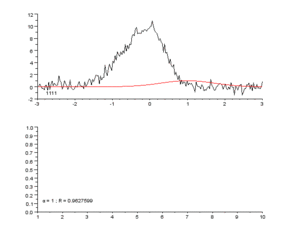# Curve fitting facts for kids

Kids Encyclopedia FactsFitting of a noisy curve by an asymmetrical peak model, with an iterative process (Gauss-Newton algorithm with variable damping factor α).
Top: raw data and model.
Bottom: evolution of the normalised sum of the squares of the errors.

Curve fitting is constructing a mathematical function which best fits a set of data points.

Curve fitting may involve either interpolation or smoothing. Using interpolation requires an exact fit to the data. With smoothing, a "smooth" function is constructed, that fit the data approximately. A related topic is regression analysis, which focuses more on questions of statistical inference such as how much uncertainty is present in a curve that is fit to data observed with random errors.

Fitted curves can be used to help data visualization, to guess values of a function where no data is available, and to summarize the relationships among two or more variables. Extrapolation refers to the use of a fitted curve beyond the range of the observed data. This is subject to a degree of uncertainty, since it may reflect the method used to construct the curve as much as it reflects the observed data.

## Images for kidsCurve fitting Facts for Kids. Kiddle Encyclopedia.